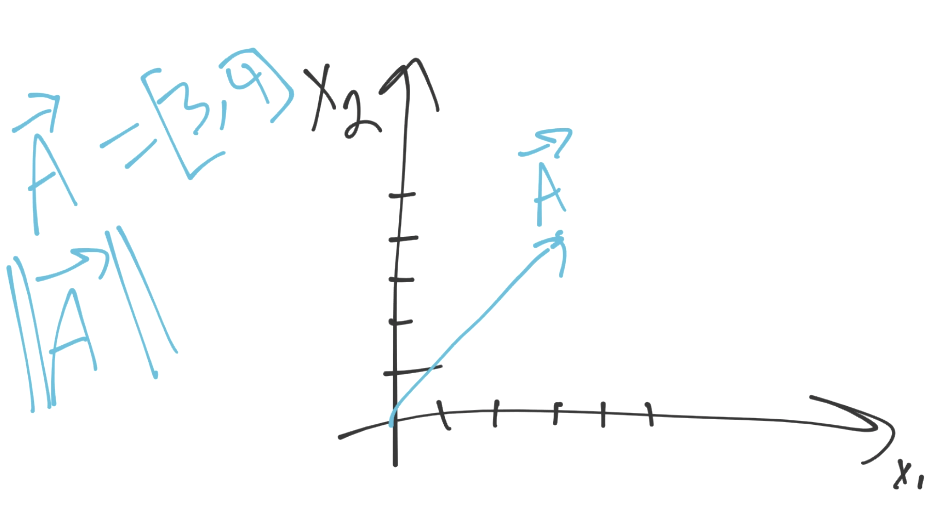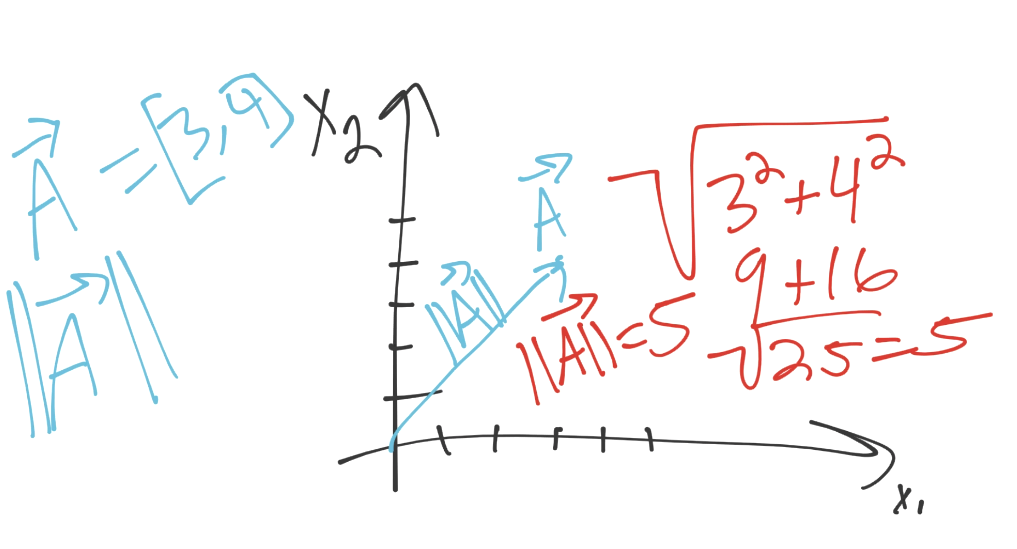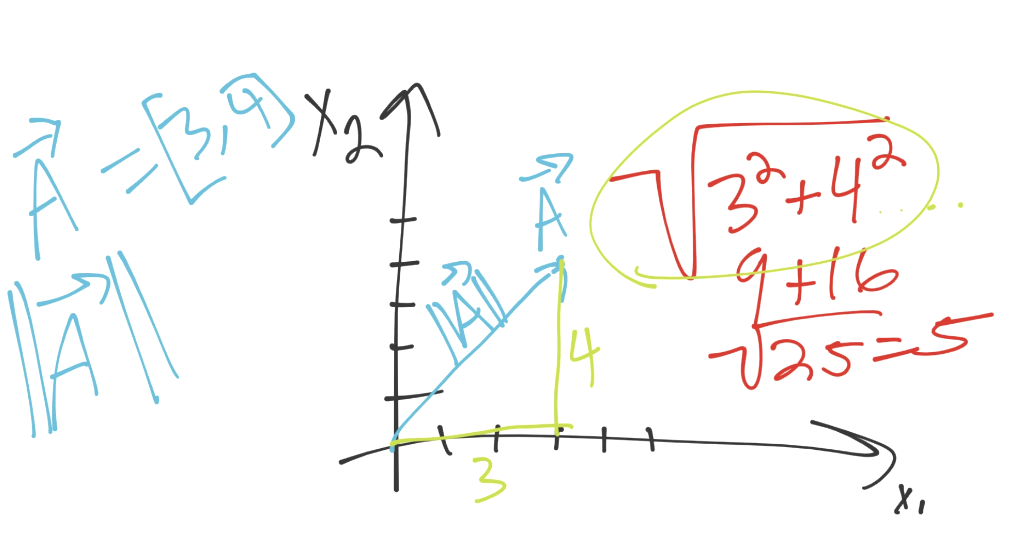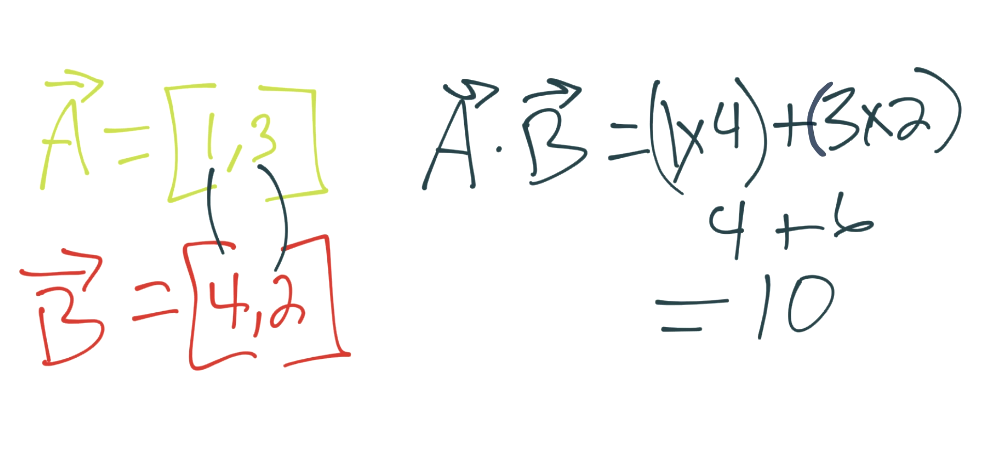## Vector Basics

Welcome to the 21th part of our machine learning tutorial series and the next part in our Support Vector Machine section. In this tutorial, we're going to be covering some of the basics of vectors, which are integral to the concepts of the Support Vector Machine.

First, a vector has both a magnitude and a direction:In the above example, vector A (denoted with an arrow above it), is moving towards [3,4]. Think of each "coordinate" as movement in that "dimension." In our case, we have two dimensions. We're moving 3 units in dimension 1, and 4 units in dimension 2. That's the direction, what's the magnitude? That's something we've already seen before, which is Euclidean distance, the norm, or the magnitude, it's all the same. Most importantly to us, the calculation for all of it is the same for us (the square root of the squared, and summed, constituents).In our case, the magnitude of a is 5. If you look at the graph as well, you might notice something else:Looks a whole lot like Pythagorean's Theorem for a triange's hypotenuse! It is indeed the same formula, only we're possibly going to be going into many more dimensions, and there wont be a simple triangle anymore.

Simple enough, next up: Dot Product. What happens when we do the vector dot product? Let's say we have two vectors, A and B. A is [1,3] and vector B is [4,2]. What we do is take each matching placed value and mutliply them together, then sum all of those. For example:Alright, now that we have those things down, we're ready to move on to the Support Vector Machine itself, first off will be some of the assertions we, the scientist, are making on the machine.

The next tutorial:• Practical Machine Learning Tutorial with Python Introduction

• Regression - Intro and Data

• Regression - Features and Labels

• Regression - Training and Testing

• Regression - Forecasting and Predicting

• Pickling and Scaling

• Regression - Theory and how it works

• Regression - How to program the Best Fit Slope

• Regression - How to program the Best Fit Line

• Regression - R Squared and Coefficient of Determination Theory

• Regression - How to Program R Squared

• Creating Sample Data for Testing

• Classification Intro with K Nearest Neighbors

• Applying K Nearest Neighbors to Data

• Euclidean Distance theory

• Creating a K Nearest Neighbors Classifer from scratch

• Creating a K Nearest Neighbors Classifer from scratch part 2

• Testing our K Nearest Neighbors classifier

• Final thoughts on K Nearest Neighbors

• Support Vector Machine introduction

• Vector Basics
• Support Vector Assertions

• Support Vector Machine Fundamentals

• Constraint Optimization with Support Vector Machine

• Beginning SVM from Scratch in Python

• Support Vector Machine Optimization in Python

• Support Vector Machine Optimization in Python part 2

• Visualization and Predicting with our Custom SVM

• Kernels Introduction

• Why Kernels

• Soft Margin Support Vector Machine

• Kernels, Soft Margin SVM, and Quadratic Programming with Python and CVXOPT

• Support Vector Machine Parameters

• Machine Learning - Clustering Introduction

• Handling Non-Numerical Data for Machine Learning

• K-Means with Titanic Dataset

• K-Means from Scratch in Python

• Finishing K-Means from Scratch in Python

• Hierarchical Clustering with Mean Shift Introduction

• Mean Shift applied to Titanic Dataset

• Mean Shift algorithm from scratch in Python

• Dynamically Weighted Bandwidth for Mean Shift

• Introduction to Neural Networks

• Installing TensorFlow for Deep Learning - OPTIONAL

• Introduction to Deep Learning with TensorFlow

• Deep Learning with TensorFlow - Creating the Neural Network Model

• Deep Learning with TensorFlow - How the Network will run

• Deep Learning with our own Data

• Simple Preprocessing Language Data for Deep Learning

• Training and Testing on our Data for Deep Learning

• 10K samples compared to 1.6 million samples with Deep Learning

• How to use CUDA and the GPU Version of Tensorflow for Deep Learning

• Recurrent Neural Network (RNN) basics and the Long Short Term Memory (LSTM) cell

• RNN w/ LSTM cell example in TensorFlow and Python

• Convolutional Neural Network (CNN) basics

• Convolutional Neural Network CNN with TensorFlow tutorial

• TFLearn - High Level Abstraction Layer for TensorFlow Tutorial

• Using a 3D Convolutional Neural Network on medical imaging data (CT Scans) for Kaggle

• Classifying Cats vs Dogs with a Convolutional Neural Network on Kaggle

• Using a neural network to solve OpenAI's CartPole balancing environment